# Banking System Project using C++

## Banking System Project using C++:

In this article, we are going to develop Banking System Project using C++ Language. It is a project for banking providing the minimal features of the banking system. We can perform the following tasks in this project.

1. Create an Account
2. Check Balance in a particular Account
3. Deposit or withdraw cash from a particular Account
4. Close any particular Account
5. List all the Accounts

Let us first perform all these tasks and then we will explain to you the program code.

##### Create Account: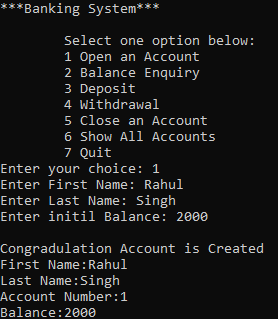Here we have chosen option ‘1’ from the menu to open an account. Then we have entered the first and last name of the user i.e. “Rahul Singh”. Then we have given the initial balance as 2000. After providing the details, we got the message “Congratulation Account is created” and the details of the user will be printed on the screen.

##### Create Another Account: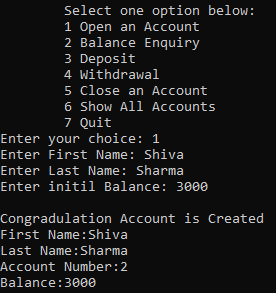Here we have created another account of the name “Shiva Sharma” with an initial balance of 3000. This is the 2nd account that we have created so the account number is 2. In the same way, we can create as many accounts as we want.

##### Check Account Balance: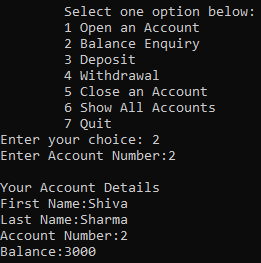We have chosen option ‘2’ which is “Balance Enquiry”. To check the balance in a particular account we have to provide the account number. We have given the account number as 2. The details of account number 2 will be displayed on the screen.

##### Deposit Money to an Account: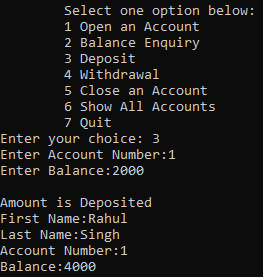The 3rd option is the deposit. To deposit in a bank account, we should know the account number and balance that we want to deposit. Here we have given account number 1 and the balance as 2000. The details of that account will be shown on the screen with an updated balance.

##### Withdraw Money from an Account: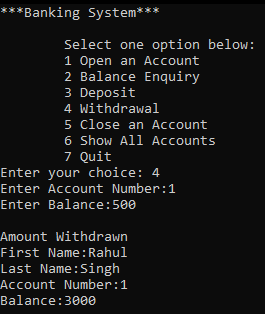4th option is withdrawal from an account. Here we need the account number and the withdrawal balance.

##### Close Account: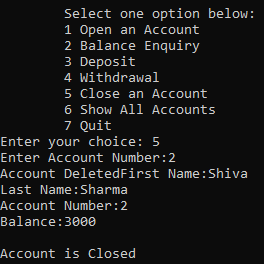The 5th option is to close an account. Here we need to provide the account number only. Then the account details will be displayed and we got the message “Account is closed”.

##### List all Accounts with Details: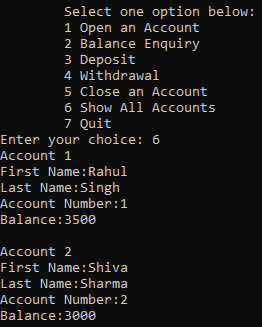6th option is to list all the accounts that we have created. This will display all the accounts with their details on the screen.

##### Quit Application:7th option is to quit. This will close our Banking program. Now let us understand the code of this project.

##### Working Procedure of Banking System Project using C++:

In this project, we have written some classes for different purposes and the main function. We have taken a constant MIN_BALANCE of value 500. Then we have a class InsufficientFunds which will act as an exception for insufficient funds.

Next, we created a class Account. This class is having accountNumber, firstName, lastName, balance, and NextAccountNumber (of type static). If you want more details then you can add more variables. Then we have defined the default constructor and parameterized constructor for class Account. Then we have some getters or accessors i.e. getAccNo (), getFirstName () etc. We haven’t written mutators because all these things cannot be modified. Once the account number is assigned it cannot be changed. And the first name and last name of a person cannot be changed associated with that account.

Next, we have defined functions for deposit and withdrawal. Then for accessing the static member that is NextAccountNumber, we have created two static functions that are setLastAccountNumber () and getLastAccountNumber (). We are using files for storing the data. If we quit the program then we will start again then Account should know what was the last account number and what should be the next account number.

Then for saving in the file and retrieving from the file, we have created friend functions. Also, to display the details of the Account, we have an overloaded insertion operator which is ostream operator.

Next, we created a class Bank. Bank will have a collection of Accounts so we have created an object accounts of type map. Because we are searching based on the account number so we have taken a map. The first type of map is the account number and the second is the Account class itself. This map container is useful for storing all the accounts. So when the program starts it should get all the account details from the file and store them.

So, when we will be using the application then the application will be accessing the columns. Then we have defined all the necessary functions for this class and some functions we have defined outside the class. So, for complete understanding, you have to read the above program and code it by yourself.

##### Banking System Project Code using C++
```#include <iostream>
#include <fstream>
#include <cstdlib>
#include <vector>
#include<map>
using namespace std;

#define MIN_BALANCE 500

class InsufficientFunds
{
};

class Account
{
private:
long accountNumber;
string firstName;
string lastName;
float balance;
static long NextAccountNumber;

public:
Account()
{
}
Account(string fname, string lname, float balance);
long getAccNo()
{
return accountNumber;
}
string getFirstName()
{
return firstName;
}
string getLastName()
{
return lastName;
}
float getBalance()
{
return balance;
}
void Deposit(float amount);
void Withdraw(float amount);
static void setLastAccountNumber(long accountNumber);
static long getLastAccountNumber();
friend ofstream & operator << (ofstream & ofs, Account & acc);
friend ifstream & operator >> (ifstream & ifs, Account & acc);
friend ostream & operator << (ostream & os, Account & acc);
};

long Account::NextAccountNumber = 0;
class Bank
{
private:
map < long, Account > accounts;

public:
Bank();
Account OpenAccount(string fname, string lname, float balance);
Account BalanceEnquiry(long accountNumber);
Account Deposit(long accountNumber, float amount);
Account Withdraw(long accountNumber, float amount);
void CloseAccount(long accountNumber);
void ShowAllAccounts();
~Bank();
};

int main()
{
Bank b;
Account acc;
int choice;
string fname, lname;
long accountNumber;
float balance;
float amount;

cout << "***Banking System***" << endl;
do
{
cout << "\n\tSelect one option below:";
cout << "\n\t1 Open an Account";
cout << "\n\t2 Balance Enquiry";
cout << "\n\t3 Deposit";
cout << "\n\t4 Withdrawal";
cout << "\n\t5 Close an Account";
cout << "\n\t6 Show All Accounts";
cout << "\n\t7 Quit";
cout << "\nEnter your choice: ";
cin >> choice;
switch (choice)
{
case 1:
cout << "Enter First Name: ";
cin >> fname;
cout << "Enter Last Name: ";
cin >> lname;
cout << "Enter initil Balance: ";
cin >> balance;
acc = b.OpenAccount (fname, lname, balance);
cout << endl << "Congradulation Account is Created" << endl;
cout << acc;
break;

case 2:
cout << "Enter Account Number:";
cin >> accountNumber;
acc = b.BalanceEnquiry (accountNumber);
cout << endl << "Your Account Details" << endl;
cout << acc;
break;

case 3:
cout << "Enter Account Number:";
cin >> accountNumber;
cout << "Enter Balance:";
cin >> amount;
acc = b.Deposit (accountNumber, amount);
cout << endl << "Amount is Deposited" << endl;
cout << acc;
break;

case 4:
cout << "Enter Account Number:";
cin >> accountNumber;
cout << "Enter Balance:";
cin >> amount;
acc = b.Withdraw (accountNumber, amount);
cout << endl << "Amount Withdrawn" << endl;
cout << acc;
break;

case 5:
cout << "Enter Account Number:";
cin >> accountNumber;
b.CloseAccount (accountNumber);
cout << endl << "Account is Closed" << endl;
cout << acc;

case 6:
b.ShowAllAccounts ();
break;

case 7:
break;

default:
cout << "\nEnter corret choice";
exit (0);
}
}
while (choice != 7);
return 0;
}

Account::Account (string fname, string lname, float balance)
{
NextAccountNumber++;
accountNumber = NextAccountNumber;
firstName = fname;
lastName = lname;
this->balance = balance;
}

void Account::Deposit (float amount)
{
balance += amount;
}

void Account::Withdraw (float amount)
{
if (balance - amount < MIN_BALANCE)
throw InsufficientFunds ();
balance -= amount;
}

void Account::setLastAccountNumber (long accountNumber)
{
NextAccountNumber = accountNumber;
}

long Account::getLastAccountNumber ()
{
return NextAccountNumber;
}

ofstream & operator << (ofstream & ofs, Account & acc)
{
ofs << acc.accountNumber << endl;
ofs << acc.firstName << endl;
ofs << acc.lastName << endl;
ofs << acc.balance << endl;
return ofs;
}

ifstream & operator >> (ifstream & ifs, Account & acc)
{
ifs >> acc.accountNumber;
ifs >> acc.firstName;
ifs >> acc.lastName;
ifs >> acc.balance;
return ifs;
}

ostream & operator << (ostream & os, Account & acc)
{
os << "First Name:" << acc.getFirstName () << endl;
os << "Last Name:" << acc.getLastName () << endl;
os << "Account Number:" << acc.getAccNo () << endl;
os << "Balance:" << acc.getBalance () << endl;
return os;
}

Bank::Bank ()
{
Account account;
ifstream infile;
infile.open ("Bank.data");
if (!infile)
{
return;
}
while (!infile.eof ())
{
infile >> account;
accounts.insert (pair < long, Account > (account.getAccNo (), account));
}
Account::setLastAccountNumber (account.getAccNo ());
infile.close ();
}

Account Bank::OpenAccount (string fname, string lname, float balance)
{
ofstream outfile;
Account account (fname, lname, balance);
accounts.insert (pair < long, Account > (account.getAccNo (), account));
outfile.open ("Bank.data", ios::trunc);
map < long, Account >::iterator itr;
for (itr = accounts.begin (); itr != accounts.end (); itr++)
{
outfile << itr->second;
}
outfile.close ();
return account;
}

Account Bank::BalanceEnquiry (long accountNumber)
{
map < long, Account >::iterator itr = accounts.find (accountNumber);
return itr->second;
}

Account Bank::Deposit (long accountNumber, float amount)
{
map < long, Account >::iterator itr = accounts.find (accountNumber);
itr->second.Deposit (amount);
return itr->second;
}

Account Bank::Withdraw (long accountNumber, float amount)
{
map < long, Account >::iterator itr = accounts.find (accountNumber);
itr->second.Withdraw (amount);
return itr->second;
}

void Bank::CloseAccount (long accountNumber)
{
map < long, Account >::iterator itr = accounts.find (accountNumber);
cout << "Account Deleted" << itr->second;
accounts.erase (accountNumber);
}

void Bank::ShowAllAccounts ()
{
map < long, Account >::iterator itr;
for (itr = accounts.begin (); itr != accounts.end (); itr++)
{
cout << "Account " << itr->first << endl << itr->second << endl;
}
}

Bank::~Bank ()
{
ofstream outfile;
outfile.open ("Bank.data", ios::trunc);
map < long, Account >::iterator itr;
for (itr = accounts.begin (); itr != accounts.end (); itr++)
{
outfile << itr->second;
}
outfile.close ();
}
```

Here, in this article, we developed Banking System Project using C++ and I hope you enjoy this Banking System Project using C++ Language article.